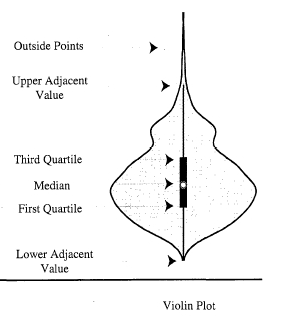# Violin Plot Using Seaborn

Manav Narula May 13, 2021

The `violinplot()` function creates such a graph and depicts the distribution like a combination between kernel density graph and a boxplot. It is heavily used by analytics and statisticians to understand the distribution of categorical data. Its main advantage over the traditional boxplots is that they can be used for distributions with multiple peaks also.

The violin figure in the plot can be a little difficult to understand, but it can depict different statistical values. The following figure will explain this.Apart from DataFrames, the `violinplot()` function can work with a single series object, numpy array, or a list vector.

In the following example, we will plot the violin plot distribution of a single variable.

``````import random
import numpy as np
n = random.sample(range(0,50),30)
arr = np.array(n)
sns.violinplot(n)
``````Note that the sample size has a huge impact on the final figure in the violin plot. The plot can be misleading for a small sample size. If this is still a little confusing, one can plot a simple scatter plot over the violin plot to get a better understanding.

For example,

``````import random
import numpy as np
n = random.sample(range(0,50),30)
arr = np.array(n)
sns.violinplot(n)
sns.stripplot(n, color = 'red')
``````The `stripplot()` function here plots a scatter plot over the violin plot. Similarly, you can also plot a box plot over the violin plot to understand the similarity and differences between both of them.

The `violinplot()` main use lies with datasets, showing distributions of data with multiple categories. In the below code, we will implement this.

``````import pandas as pd
import matplotlib.pyplot as plt
import seaborn as sns

df = pd.DataFrame({"Quantity": [5,6,7,8,5,6,7,8,5,6,7,8,5,6,7,8],
"Price": [9,10,15,16,13,14,15,18,11,12,14,15,16,17,18,19],
"Day" : [1,1,1,1,1,1,1,1,2,2,2,2,2,2,2,2],
"Product": ['A','A','A','A','B','B','B','B',
'A','A','A','A','B','B','B','B']})

sns.violinplot(data = df, y = "Price", x = "Quantity", hue = "Product")
``````Notice how we were able to efficiently plot the distributions of the price of two different products at different quantities on two days. Notice the use of the `hue` parameter to plot two products with different colors.

A lot of other arguments can be used to tweak and modify the final figure. We can use the `split` parameter to compare the categories easily. Their order can be managed using the `order` parameter. Each observation can be shown inside the plot using the `inner` parameter.

In the code below, we try to use some of these arguments.

``````import pandas as pd
import matplotlib.pyplot as plt
import seaborn as sns

df = pd.DataFrame({"Quantity": [5,6,7,8,5,6,7,8,5,6,7,8,5,6,7,8],
"Price": [9,10,15,16,13,14,15,18,11,12,14,15,16,17,18,19],
"Day" : [1,1,1,1,1,1,1,1,2,2,2,2,2,2,2,2],
"Product": ['A','A','A','A','B','B','B','B',
'A','A','A','A','B','B','B','B']})

sns.violinplot(data = df, y = "Price", x = "Quantity", hue = "Product", inner = 'stick', split = True)
``````The `violinplot()` function returns a matplotlib axes-type object to use all such matplotlib functions to customize the final figure. If we want to return a `FacetGrid` type object, we can use the `catplot()` function and specify the `kind` parameter as `violin`.

Author: Manav Narula

Manav is a IT Professional who has a lot of experience as a core developer in many live projects. He is an avid learner who enjoys learning new things and sharing his findings whenever possible.